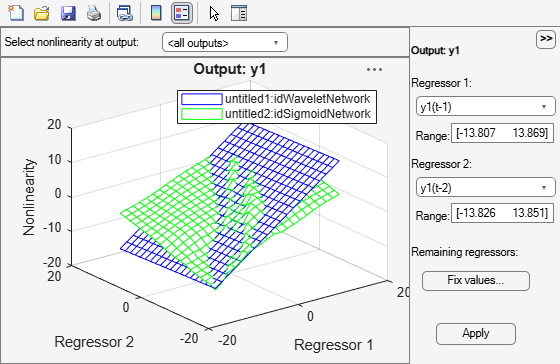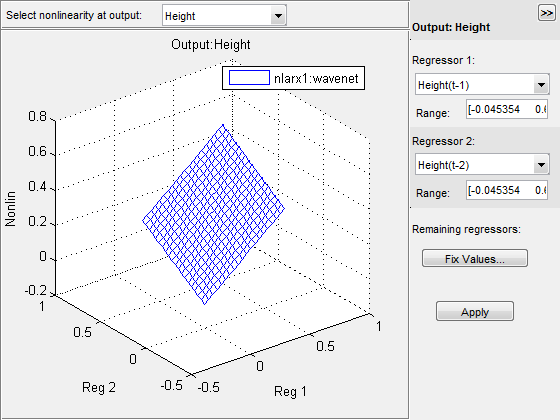Documentation

idnlarx/plot

Plot nonlinearity of nonlinear ARX model

Description

example

plot(model) plots the nonlinearity of a nonlinear ARX model on a nonlinear ARX plot. The plot shows the nonlinearity for all outputs of the model as a function of its input regressors.

plot(model,color) specifies the color to use.

plot(model1,...,modelN) generates the plot for multiple models.

example

plot(model1,color1...,modelN,colorN) specifies the color for each model. You do not need to specify the color for all models.

example

plot(___,'NumberofSamples',N) specifies the number of samples to use to grid the regressor space on each axis. This syntax can include any of the input argument combinations in the previous syntaxes.

Examples

collapse all

Estimate a nonlinear ARX model and plot its nonlinearity.

model1 = nlarx(z1,[4 2 1],'wave','nlr',[1:3]);
plot(model1)In the plot window, you can choose:

• The regressors to use on the plot axes, and specify the center points for the other regressors in the configuration panel. For multi-output models, each output is plotted separately.

• The output to view from the drop-down list located at the top of the plot.

model1 = nlarx(z1,[4 2 1],'wave','nlr',[1:3]);
model2 = nlarx(z1,[4 2 1],'sigmoidnet','nlr',[1:3]);
plot(model1,'b', model2, 'g')model1 = nlarx(z1,[4 2 1],'wave','nlr',[1:3]);
model2 = nlarx(z1,[4 2 1],'sigmoidnet','nlr',[1:3]);
plot(model1,'b', model2, 'g','NumberofSamples',50)Input Arguments

collapse all

Estimated nonlinear ARX model, specified as an idnlarx model object. Use nlarx to estimate the model.

Color to use to plot the regressors, specified as one of the following:

• Character vector of color name, specified as one of the following:

• 'b'

• 'y'

• 'm'

• 'c'

• 'r'

• 'g'

• 'w'

• 3-element double vector of RGB values

By default, the colors are automatically chosen.

Data Types: double | char

Number of points used on the regressor axis to display the regressor samples, specified as a positive integer.

Data Types: double

collapse all

What is a Nonlinear ARX Plot?

A nonlinear ARX plot displays the evaluated model nonlinearity for a chosen model output as a function of one or two model regressors. For a model M, the model nonlinearity (M.Nonlinearity) is a nonlinearity estimator function, such as wavenet, sigmoidnet, or treepartition, that uses model regressors as its inputs.To understand what is plotted, suppose that {r1,r2,…,rN} are the N regressors used by a nonlinear ARX model M with nonlinearity nl corresponding to a model output. You can use getreg(M) to view these regressors. The expression Nonlin = evaluate(nl,[v1,v2,...,vN]) returns the model output for given values of these regressors, that is, r1 = v1, r2 = v2, ..., rN = vN. For plotting the nonlinearities, you select one or two of the N regressors, for example, rsub = {r1,r4}. The software varies the values of these regressors in a specified range, while fixing the value of the remaining regressors, and generates the plot of Nonlin vs. rsub. By default, the software sets the values of the remaining fixed regressors to their estimated means, but you can change these values. The regressor means are stored in the Nonlinearity.Parameters.RegressorMean property of the model.

Examining a nonlinear ARX plot can help you gain insight into which regressors have the strongest effect on the model output. Understanding the relative importance of the regressors on the output can help you decide which regressors to include in the nonlinear function for that output. If the shape of the plot looks like a plane for all the chosen regressor values, then the model is probably linear in those regressors. In this case, you can remove the corresponding regressors from nonlinear block, and repeat the estimation.

Furthermore, you can create several nonlinear models for the same data using different nonlinearity estimators, such a wavenet network and treepartition, and then compare the nonlinear surfaces of these models. Agreement between plots for various models increases the confidence that these nonlinear models capture the true dynamics of the system.

To learn more about configuring the plot, see Configuring a Nonlinear ARX Plot.